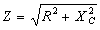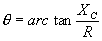## Most Used Formulas (cont.)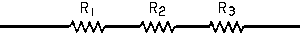of resistance in series,                       Z = R1 + R2 + R3 ... etc.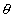= 0o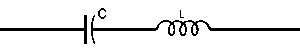of inductance and capacitance in series,                        Z = XL - XC= -90o when XL < XC                         = 0o when XL = XC                         = +90o when XL > XC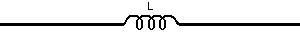of inductance alone                       Z = XL= +90o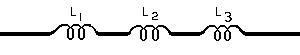of inductance in series,                      Z = XL1 + XL2 + XL3 ... etc.= +90oof resistance, inductance and capacitance in series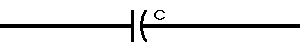of capacitance alone,                       Z = XC= -90o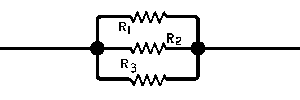of resistance in parallel,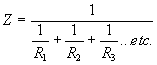= 0o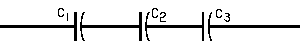of capacitance and resistance in parallel,                      Z = XC1 + XC2 + XC3 ...etc.= -90o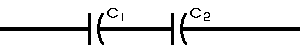or where only 2 capacitances C1 and C2 are  involved,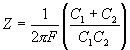= -90o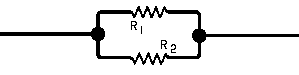of resistance in parallel,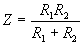= 0o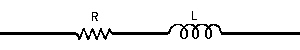of resistance and inductance in series,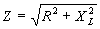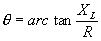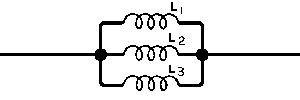of inductance in parallel,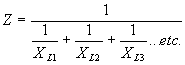= +90oof resistance and capacitance in series,